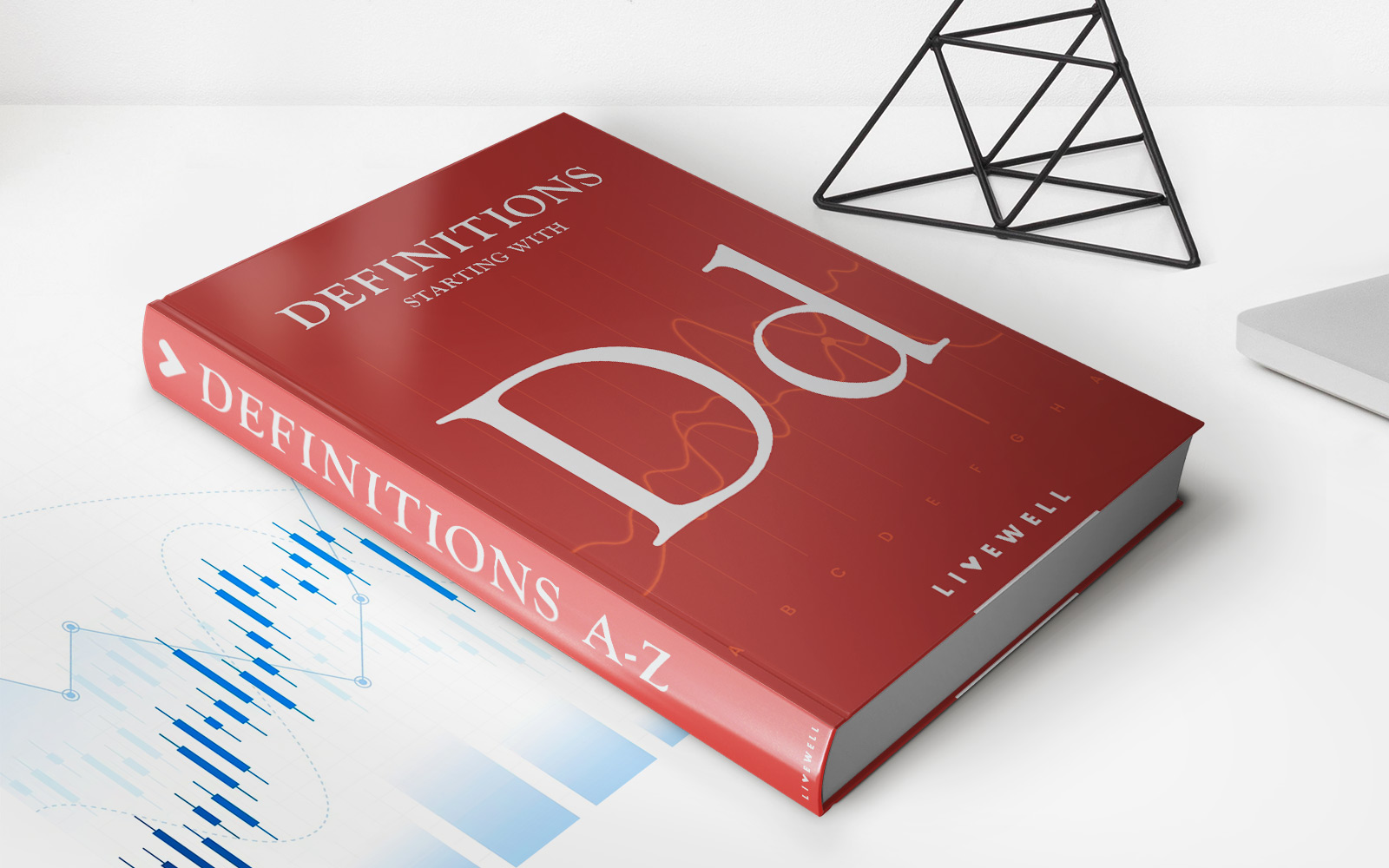Home>Finance>Dutch Book Theorem DefinitionFinance

# Dutch Book Theorem Definition

Learn the definition of the Dutch Book Theorem in the field of Finance. Discover how this concept impacts financial markets and decision-making.

## The Dutch Book Theorem: Understanding its Definition and Significance in Finance

When it comes to financial strategies, investors and traders are constantly seeking methods to maximize their returns while minimizing risk. One such concept that plays a crucial role in finance is the Dutch Book Theorem. In this blog post, we will dive into its definition, explore its significance in the world of finance, and discuss how it can impact decision-making in investment scenarios.

## Key Takeaways:

• The Dutch Book Theorem is a concept used in finance to determine the rationality of a set of odds or probabilities.
• According to the theorem, if a set of odds violates certain conditions, a rational individual could potentially be guaranteed a monetary loss.

## Understanding the Dutch Book Theorem

The Dutch Book Theorem, also known as the Dutch Book Argument, is a concept that stems from probability and decision theory. It provides a framework to assess the rationality of odds or probabilities when making financial decisions.

At its core, the Dutch Book Theorem states that if a set of odds or probabilities violates specific conditions, there exists an opportunity for a rational individual to create a series of bets that guarantee a monetary gain, regardless of the actual outcome.

Let’s break down the conditions that need to be met for a Dutch Book situation:

1. No Arbitrage: There should be no possibility to execute a series of risk-free trades that result in a net profit.
2. Coherence: The set of odds or probabilities should adhere to the principles of rational decision-making, such as transitivity and sure thing principle.
3. Market Prices: The odds or probabilities should be reasonably priced in the market, reflecting the actual likelihood of the events occurring.

When one or more of these conditions are violated, it implies that there is an opportunity for a “Dutch Book” to be made, where an individual can bet and create a combination of outcomes that guarantees a profit. This theorem is important in analyzing the efficiency and rationality of odds or probabilities provided by bookmakers, financial markets, or bettors themselves.

## Significance of the Dutch Book Theorem in Finance

The Dutch Book Theorem has significant implications in finance. Here are a few key points to consider:

• Market Efficiency: The theorem helps identify and evaluate potential inefficiencies in market prices for odds or probabilities. If a Dutch Book can be created, it highlights inconsistencies that can be exploited by savvy investors.
• Assessing Rationality: By applying the Dutch Book Theorem, financial individuals can determine if their own odds or probabilities align with the principles of rational decision-making. It serves as a tool to evaluate the coherence and consistency of one’s own beliefs.
• Portfolio Management: The theorem aids in constructing robust portfolios by considering the rationality of odds or probabilities associated with investment decisions. By avoiding Dutch Book opportunities, investors can enhance their long-term performance.

In conclusion, the Dutch Book Theorem is an essential concept in finance that evaluates the rationality of odds or probabilities. By adhering to specific conditions, this theorem helps market participants identify potential inefficiencies and make more informed decisions. Understanding and applying the Dutch Book Theorem can provide individuals with valuable insights into the world of finance, enabling them to optimize their strategies and mitigate risks.

• https://livewell.com/finance/dutch-book-theorem-definition/